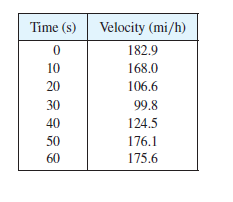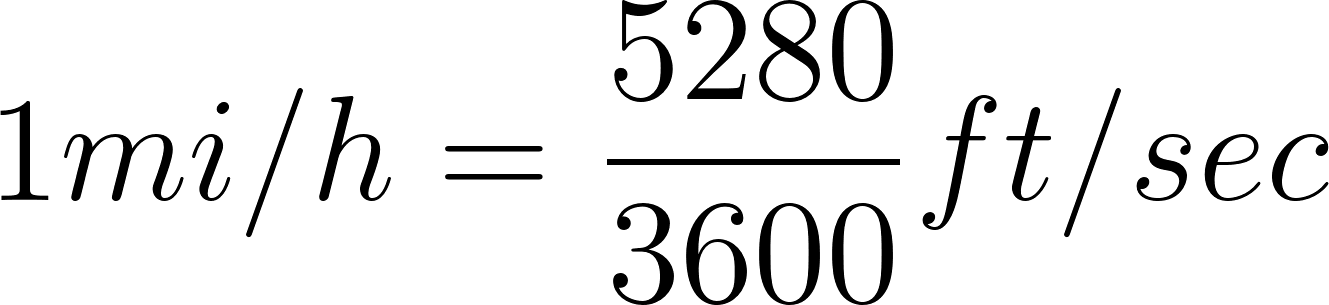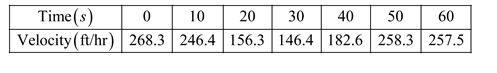×

×

# The table shows speedometer readings at 10-second intervals during a 1-minute period forISBN: 9781305270336 484

## Solution for problem 14 Chapter 5.1

Single Variable Calculus: Early Transcendentals | 8th Edition

• Textbook Solutions
• 2901 Step-by-step solutions solved by professors and subject experts
• Get 24/7 help from StudySoup virtual teaching assistantsSingle Variable Calculus: Early Transcendentals | 8th Edition

4 5 1 241 Reviews
26
3
Problem 14

The table shows speedometer readings at 10-second intervals during a 1-minute period for a car racing at the Daytona International Speedway in Florida. (a) Estimate the distance the race car traveled during this time period using the velocities at the beginning of the time intervals. (b) Give another estimate using the velocities at the end of the time periods. (c) Are your estimates in parts (a) and (b) upper and lower estimates? Explain.

Step-by-Step Solution:

Problem 14

The table shows speedometer readings at 10-second intervals during a 1-minute period for a car racing at the Daytona International Speedway in Florida. (a) Estimate the distance the race car traveled during this time period using the velocities at the beginning of the time intervals. (b) Give another estimate using the velocities at the end of the time periods. (c) Are your estimates in parts (a) and (b) upper and lower estimates? Explain.Step-by step solution

Step 1 of  4

a)

The objective is to determine distance travelled by race car using the velocities at the beginning of the intervals.

Readings of the speedometer of a car are shown below:To have the velocity in consistent units,convert the velocity in ft/s.Rewrite  the table as follows,Step 2 of 4

Step 3 of 4

##### ISBN: 9781305270336

The answer to “The table shows speedometer readings at 10-second intervals during a 1-minute period for a car racing at the Daytona International Speedway in Florida. (a) Estimate the distance the race car traveled during this time period using the velocities at the beginning of the time intervals. (b) Give another estimate using the velocities at the end of the time periods. (c) Are your estimates in parts (a) and (b) upper and lower estimates? Explain.” is broken down into a number of easy to follow steps, and 73 words. The full step-by-step solution to problem: 14 from chapter: 5.1 was answered by , our top Calculus solution expert on 03/19/18, 03:29PM. Single Variable Calculus: Early Transcendentals was written by and is associated to the ISBN: 9781305270336. This full solution covers the following key subjects: . This expansive textbook survival guide covers 95 chapters, and 5427 solutions. Since the solution to 14 from 5.1 chapter was answered, more than 2319 students have viewed the full step-by-step answer. This textbook survival guide was created for the textbook: Single Variable Calculus: Early Transcendentals, edition: 8.

Unlock Textbook Solution